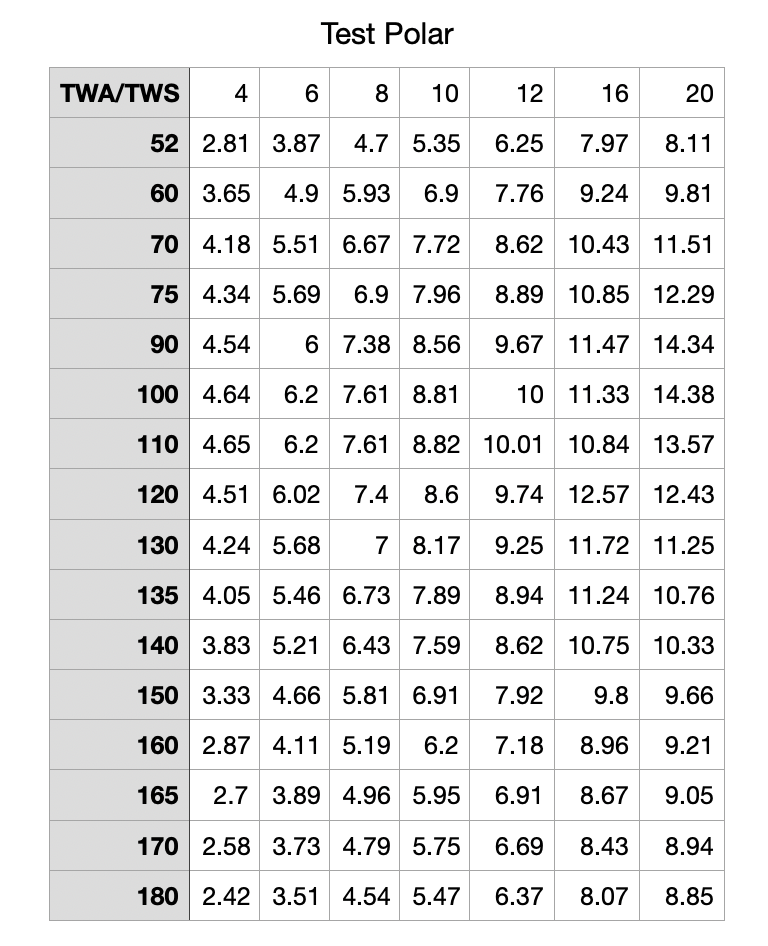#### Index

RoutingPurchaseContactLuckGribOffshore

#### Index

User Manual | Performance (Polars) | Importing a sailing polar | Spreadsheet data

When polar data is represented in a spreadsheet, such as Numbers, each column of the spreadsheet represents a true wind speed and each row represents a true wind angle.

For example:Note that the first row of this spreadsheet defines the true wind speed values represented. The text in the first row and column is ignored.

Each row, after the first one, defines a true wind angle in its first column, and then for every true wind speed column, defines the boat speed for that wind angle / speed combination.

For example, the table above specifies, that when the true wind speed is 12 knots, and the true wind angle is 90 (directly on the beam on port or starboard) that the boat speed is 9.67 knots.